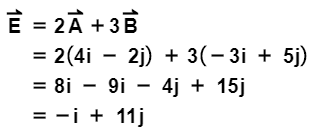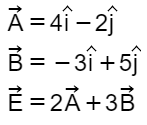# Problem: LetWhat is the direction of vector E⃗?

###### FREE Expert Solution

Vector direction:

$\overline{){\mathbf{tan}}{\mathbf{\theta }}{\mathbf{=}}\frac{\mathbf{y}}{\mathbf{x}}}$100% (243 ratings)###### Problem Details

LetWhat is the direction of vector E⃗?

Frequently Asked Questions

What scientific concept do you need to know in order to solve this problem?

Our tutors have indicated that to solve this problem you will need to apply the Unit Vectors concept. You can view video lessons to learn Unit Vectors. Or if you need more Unit Vectors practice, you can also practice Unit Vectors practice problems.